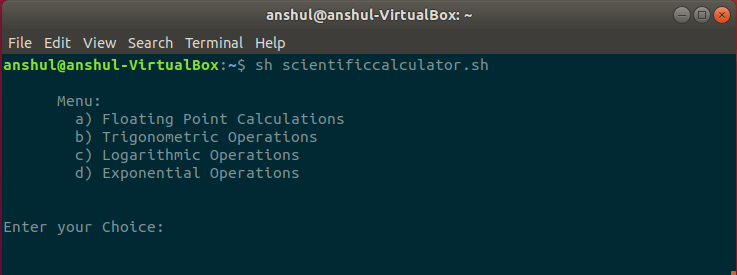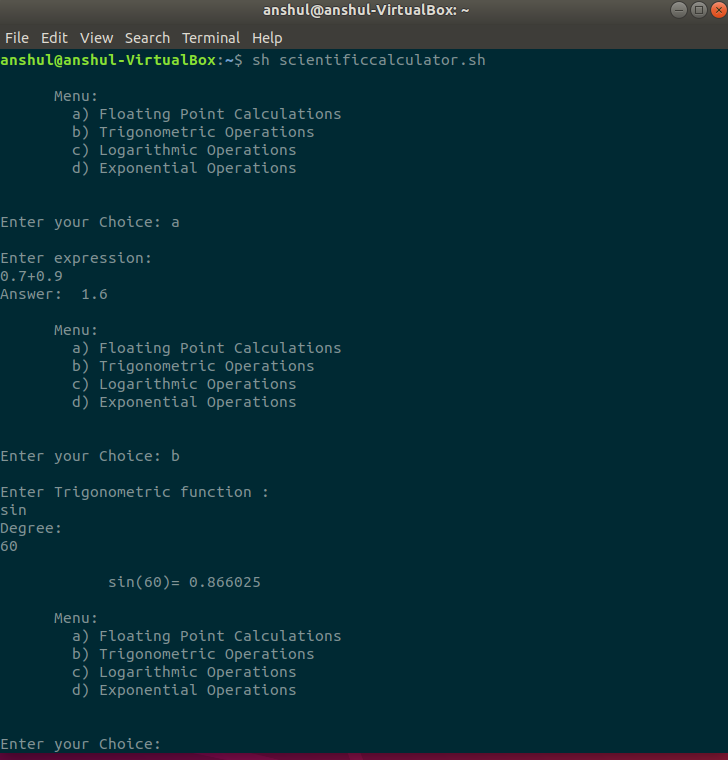# Scientific Calculator Using awk Command in Linux

The awk command in Linux allows the user to use the numeric functions, variables, logical operators and string functions. Basically, it is a utility that allows the user to write small and effective programs in the form the statements. It is mostly used in pattern scanning and processing. Here, we are using the awk command to perform the following operations:

• Floating-point calculations
• Trigonometric operations
• Logarithms
• Exponent

Syntax for awk Command

```awk options 'pattern{action }' input-file > output-file
```

Program: Here, we are using Ubuntu. We are saving the below program in file named scientificcalculator.sh. To execute this program, you can use the below command:

```sh  scientificcalculator.sh
```

 `pi=`echo ``"scale=10;4*a(1)"` `| bc -l` ` ` `  `while` `true` `do` ` `  `cat << MENU ` ` `  `      ``Menu: ` `    ``a) Floating Point Calculations ` `    ``b) Trigonometric Operations ` `    ``c) Logarithmic Operations ` `    ``d) Exponential Operations ` `     `  `MENU ` `echo ' ` `Enter your Choice: \c' ` `read choice ` ` `  `case` `\$choice in ` `     `  `    ``a)  echo ``"\nEnter expression: "` `        ``read ``exp`     `        ``cal() ` `            ``awk ``"BEGIN{print \$*}"``;     ` `        ``echo ``"Answer: "` ``cal \$``exp``` ` `        ``;; ` ` `  `    ``b)  echo ``"\nEnter Trigonometric function : "` `            ``read ``exp` `            ``echo ``"Degree: "` `            ``read degree ` ` `  `         ``e=\$(awk ``"BEGIN{print \$exp(\$degree*atan2(0,-1)/180)}"``) ` `            ``echo " ` `            ``\$``exp``(\$degree)= \$e"  ` `            ``;; ` ` `  `        ``c)  echo ``"\nEnter the logarithmic value: "` `            ``read value ` `            ``echo \$value | awk ``'{printf "%11.9f\n",log(\$1)/log(10)}'`  `            ``;; ` ` `  `        ``d)  echo ``"\nEnter the base number x: "`     `        ``read x ` `        ``echo ``"Enter exponent number y: "` `        ``read y ` `        ``E=\$(echo ``"\$x 1"` `| awk ``"{print ((\$x/1)^\$y)}"``) ` `        ``echo ``"\$x^\$y = \$E"`      `            ``;;             ` `        ``*) ` `              ``break``;;     ` `          ``*) ` `              ``break``;; ` `esac ` `done `

Output:My Personal Notes arrow_drop_upCheck out this Author's contributed articles.

If you like GeeksforGeeks and would like to contribute, you can also write an article using contribute.geeksforgeeks.org or mail your article to contribute@geeksforgeeks.org. See your article appearing on the GeeksforGeeks main page and help other Geeks.

Please Improve this article if you find anything incorrect by clicking on the "Improve Article" button below.